# Finding a percentage of a total amount in a circle graph Online Quiz

Following quiz provides Multiple Choice Questions (MCQs) related to Finding a percentage of a total amount in a circle graph. You will have to read all the given answers and click over the correct answer. If you are not sure about the answer then you can check the answer using Show Answer button. You can use Next Quiz button to check new set of questions in the quiz.Q 1 - If the class election results are as given in the circle graph and if the total votes polled were 300. How many voted for Emily?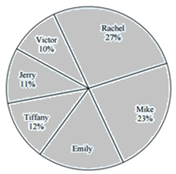### Explanation

Step 1:

Percentage of votes for Emily = 100 - 27 - 10 - 11 - 12 - 23 = 17%

Step 2:

Number of votes polled by Emily = $\frac{17}{100} \times 300 = 51$

Q 2 - If the total number of people who saw the following movies in circle graph was 220 what is the number of people who saw Dumbo?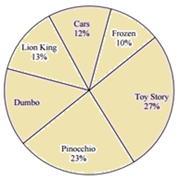### Explanation

Step 1:

Percentage of people who saw Dumbo = 100 - 27 - 10 - 13 - 12 - 23 = 15%

Step 2:

Number of people who saw Dumbo = $\frac{15}{100} \times 220 = 33$

Q 3 - The following is a circle graph showing the monthly expenditure of Jason. How much does he spend on savings as a percentage?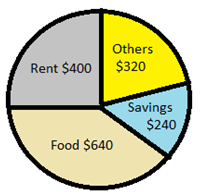### Explanation

Step 1:

Total expenditure = $400 +$640 + $320 +$240 = $1600 Amount spent on savings =$240

Step 2:

Percent of money spent on savings

= $\frac{240}{1200} \times 220 = 20\%$

Q 4 - If the circle graph gives information about the visits of 360 students to History Museum, Nature Reserve, Art Gallery etc., find the number of students who visited the History Museum?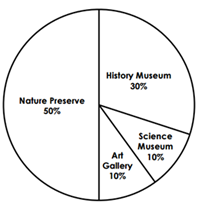### Explanation

Step 1:

Percentage of students who visited the History museum. = 30%;

Total number of students = 360

Step 2:

Number of students who visited the History museum. = $\frac{30}{100} \times 360 = 108$

Q 5 - Some of the sixth graders were surveyed about their favorite color. The circle graph shows the results of the survey. What percent and how many students like the pink color?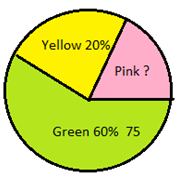### Explanation

Step 1:

Percentage of Pink

= 100% – 20% – 60% = 20%

Number of those who like green = 75

Step 2:

Percentage of those who like green = 60%

Number of those who like pink = $\frac{20}{60} \times 75 = 25$

Q 6 - The circle graph shows the number of people of different age groups, who visited a certain fast food joint during a week. What percent of the people aged below 12 years visited the food joint?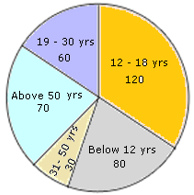### Explanation

Step 1:

Total number of people = 80 + 30 + 70 + 60 + 120 = 360

Number of people below 12 years = 80

Step 2:

Percentage of people below 12 years

= $\frac{80}{360} \times 100 = 22.22$

Q 7 - The circle graph shows the number of space vehicles sent into space during 40 years. What is the percentage of space vehicles that were sent during 1970-79?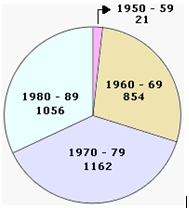### Explanation

Step 1:

Total number of space vehicles sent = 1056 + 1162 + 854 = 3072

Number of vehicles sent during 1970 - 79 = 1162

Step 2:

Percentage of vehicles sent during 1970 - 79 = $\frac{1162}{3072} \times 100 = 37.82\%$

Q 8 - The circle graph shows the percentages of items used in the making of a pizza which weighs 500 gm. Which item is used in the least quantity in the preparation of the pizza and what is its weight?### Explanation

Step 1:

Item that is used least is mushroom = 5%

Weight of the pizza = 500 gm

Step 2:

Weight of mushroom used = $\frac{5}{100} \times 500 = 25gm$

Q 9 - Mr. Nelson asked students in his class how they spent their leisure time. The responses are as shown in the circle graph. If there are 40 students in the class how many students spent their leisure time in playing?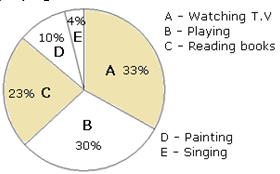### Explanation

Step 1:

The percentage of students who spent time in playing = 30%

Step 2:

Number of students spending time in playing = $\frac{30}{100} \times 40 = 12$

Q 10 - A circle graph is drawn to represent a sample of 100 people who like various sports. Find the number of people who like baseball out of a population of 800 people.### Explanation

Step 1:

Population = 800 people

Percentage of people liking baseball = 23%

Step 2:

Number of people liking baseball = $\frac{23}{100} \times 800 = 184$

finding_percentage_of_total_amount_in_circle_graph.htm